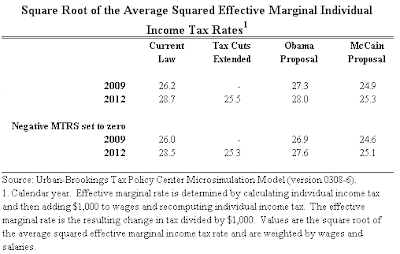## Thursday, October 02, 2008

### Obama, McCain, and Quadratic Averaging

Yesterday, I proposed a new metric for computing the average marginal tax rate. Standard theory says that the deadweight loss of taxation rises approximately with the square of the tax rate. That is, increasing a tax rate by one percentage point reduces welfare by more if the tax rate is already high than if it is low. To take this fact into account when computing the average marginal tax rate under alternative tax plans, I proposed quadratic averaging: Compute the square root of the average squared marginal tax rate.

Len Burman at the Tax Policy Center was intrigued by the the idea and quickly produced the numbers. He calls it the "Mankiw effective tax rate." (I just love that.) Here is what he finds:For example, look at the results for 2012. Under current law, the Bush tax cuts will expire, and the average marginal tax rate, calculated using my quadratic averaging, will be 28.7 percent. If the Bush tax cuts are extended, the average marginal tax rate will be 25.5 percent. The McCain plan would keep the average marginal tax rate at about this level (25.3 percent), while the Obama plan would raise the average marginal tax rate to 28.0 percent.